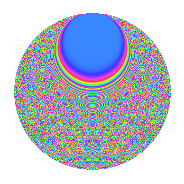# Properties

 Label 2016.2.ftLevel 2016 Weight 2 Character orbit ft Rep. character $$\chi_{2016}(37,\cdot)$$ Character field $$\Q(\zeta_{24})$$ Dimension 1264 Sturm bound 768

# Related objects

## Defining parameters

 Level: $$N$$ = $$2016 = 2^{5} \cdot 3^{2} \cdot 7$$ Weight: $$k$$ = $$2$$ Character orbit: $$[\chi]$$ = 2016.ft (of order $$24$$ and degree $$8$$) Character conductor: $$\operatorname{cond}(\chi)$$ = $$224$$ Character field: $$\Q(\zeta_{24})$$ Sturm bound: $$768$$

## Dimensions

The following table gives the dimensions of various subspaces of $$M_{2}(2016, [\chi])$$.

Total New Old
Modular forms 3136 1296 1840
Cusp forms 3008 1264 1744
Eisenstein series 128 32 96

## Trace form

 $$1264q + 4q^{2} - 4q^{4} + 4q^{5} - 8q^{7} + 16q^{8} + O(q^{10})$$ $$1264q + 4q^{2} - 4q^{4} + 4q^{5} - 8q^{7} + 16q^{8} - 4q^{10} + 4q^{11} - 16q^{13} + 40q^{14} - 8q^{16} - 4q^{19} - 16q^{20} - 24q^{22} - 4q^{23} - 4q^{25} + 4q^{26} + 12q^{28} + 16q^{29} - 56q^{31} + 4q^{32} - 32q^{34} - 16q^{35} - 4q^{37} + 44q^{38} + 60q^{40} + 16q^{41} - 36q^{44} - 4q^{46} + 40q^{50} + 20q^{52} - 12q^{53} - 16q^{55} + 48q^{56} - 36q^{58} - 28q^{59} - 4q^{61} - 136q^{64} + 8q^{65} + 4q^{67} + 36q^{68} - 44q^{70} + 80q^{71} - 4q^{73} + 76q^{74} - 16q^{76} + 8q^{77} - 8q^{80} + 36q^{82} + 96q^{83} - 56q^{85} + 4q^{86} + 40q^{88} + 4q^{89} + 40q^{91} - 48q^{92} - 44q^{94} + 8q^{95} - 32q^{97} - 8q^{98} + O(q^{100})$$

## Decomposition of $$S_{2}^{\mathrm{new}}(2016, [\chi])$$ into newform subspaces

The newforms in this space have not yet been added to the LMFDB.

## Decomposition of $$S_{2}^{\mathrm{old}}(2016, [\chi])$$ into lower level spaces

$$S_{2}^{\mathrm{old}}(2016, [\chi]) \cong$$ $$S_{2}^{\mathrm{new}}(224, [\chi])$$$$^{\oplus 3}$$$$\oplus$$$$S_{2}^{\mathrm{new}}(672, [\chi])$$$$^{\oplus 2}$$

## Hecke Characteristic Polynomials

There are no characteristic polynomials of Hecke operators in the database